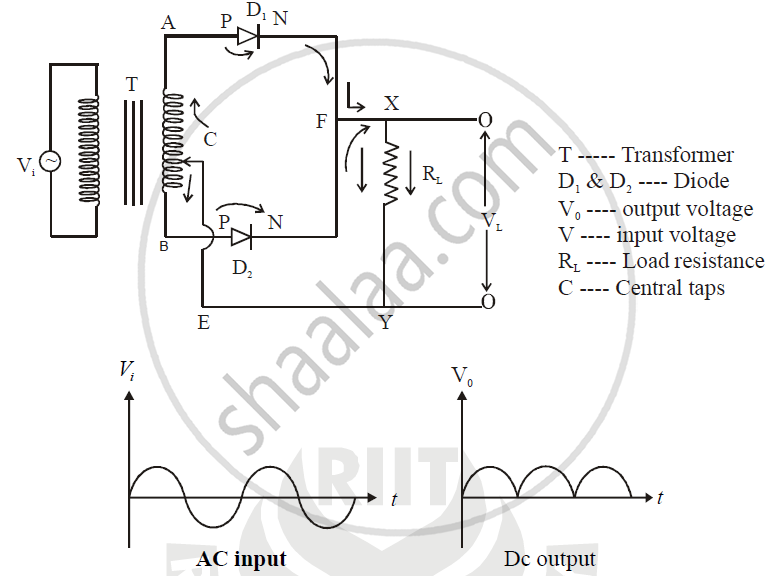ISC (Science) Class 12CISCE
Share

# Draw a Labelled Diagram of a Full Wave Rectifier. Show How Output Voltage Varies with Time If The Input Voltage is a Sinusoidal Voltage. - ISC (Science) Class 12 - Physics (Theory)

#### Question

Draw a labelled diagram of a full wave rectifier. Show how output voltage varies with time if the input voltage is a sinusoidal voltage.

#### SolutionIs there an error in this question or solution?

#### APPEARS IN

2014-2015 (March) (with solutions)
Question 15.1 | 3.00 marks

#### Video TutorialsVIEW ALL 

Solution Draw a Labelled Diagram of a Full Wave Rectifier. Show How Output Voltage Varies with Time If The Input Voltage is a Sinusoidal Voltage. Concept: Semiconductor Diode.
S